# Pearson product-moment correlation coefficient

(diff) ← Older revision | Latest revision (diff) | Newer revision → (diff)

While the modern theory of correlation and regression has its roots in the work of F. Galton, the version of the product-moment correlation coefficient in current use (2000) is due to K. Pearson [a2]. Pearson's product-moment correlation coefficientis a measure of the strength of a linear relationship between two random variablesand(cf. also Random variable) with means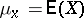,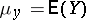and finite variances,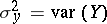: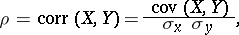where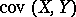is the covariance ofand,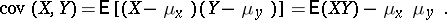It readily follows that, and thatis equal toor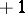if and only if each ofandis almost surely a linear function of the other, i.e.,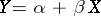() with probability(furthermore,andhave the same sign). If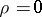,andare said to be uncorrelated. Independent random variables are always uncorrelated, however uncorrelated random variables need not be independent (cf. also Independence).

The term "product-moment" refers to the observation that, wheredenotes the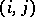th product moment ofandabout their means.

The coefficientalso plays a role in linear regression (cf. also Regression analysis). If the regression ofonis linear, then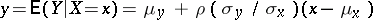, and if the regression ofonis linear, then. Note that the product of the two slopes is.

Whenandhave a bivariate normal distribution (cf. also Normal distribution),is a parameter of the joint density function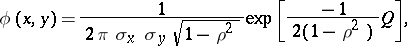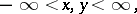with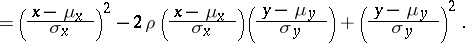Unlike the general situation, uncorrelated random variables with a bivariate normal distribution are independent.

For a random sample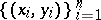from a bivariate population,is estimated by the sample correlation coefficient (cf. also Correlation coefficient), given by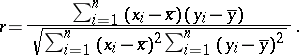If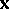and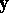denote, respectively, the vectors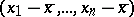and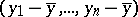, anddenotes the angle betweenand, thenFurther interpretations ofcan be found in [a3]. For details on the use ofin hypothesis testing, and for large-sample theory, see [a1].

How to Cite This Entry:
Pearson product-moment correlation coefficient. Encyclopedia of Mathematics. URL: http://encyclopediaofmath.org/index.php?title=Pearson_product-moment_correlation_coefficient&oldid=18562
This article was adapted from an original article by R.B. Nelsen (originator), which appeared in Encyclopedia of Mathematics - ISBN 1402006098. See original article